• scatter 散点图 全页折叠 语法 scatter(x,y) scatter(x,y,sz) scatter(x,y,sz,c) scatter(___,'filled') scatter(___,mkr) scatter(___,Name,Value) scatter(ax,___) s = scatter...

scatter

散点图

全页折叠

语法


scatter(x,y)


scatter(x,y,sz)


scatter(x,y,sz,c)


scatter(___,'filled')


scatter(___,mkr)


scatter(___,Name,Value)



scatter(ax,___)



s = scatter(___)


说明

示例

scatter(x,y) 在向量 x 和 y 指定的位置创建一个包含圆形的散点图。该类型的图形也称为气泡图。


示例

scatter(x,y,sz) 指定圆大小。要绘制大小相等的圆圈，请将 sz 指定为标量。要绘制大小不等的圆，请将 sz 指定为长度等于 x 和 y 的长度的向量。


示例

scatter(x,y,sz,c) 指定圆颜色。要以相同的颜色绘制所有圆圈，请将 c 指定为颜色名称或 RGB 三元组。要使用不同的颜色，请将 c 指定为向量或由 RGB 三元组组成的三列矩阵。


示例

scatter(___,'filled') 填充圆形。可以将 'filled' 选项与前面语法中的任何输入参数组合一起使用。


示例

scatter(___,mkr) 指定标记类型。


示例

scatter(___,Name,Value) 使用一个或多个名称-值对组参数修改散点图。例如，'LineWidth',2 将标记轮廓宽度设置为 2 磅。


示例

scatter(ax,___) 将在 ax 指定的坐标区中，而不是在当前坐标区中绘制图形。选项 ax 可以位于前面的语法中的任何输入参数组合之前。


示例

s = scatter(___) 返回 Scatter 对象。在创建散点图后，以后可使用 s 对其进行修改。


示例

全部折叠

创建散点图

尝试此示例

创建 x 为 0 和之间的 200 个等间距值。创建 y 为带随机干扰的余弦值。然后，创建一个散点图。


x = linspace(0,3*pi,200);
y = cos(x) + rand(1,200);
scatter(x,y)改变圆圈大小

尝试此示例

使用大小不同的圆圈创建一个散点图。以平方磅为单位指定大小


x = linspace(0,3*pi,200);
y = cos(x) + rand(1,200);
sz = linspace(1,100,200);
scatter(x,y,sz)x、y 和 sz 中的相应元素确定每个圆圈的位置和大小。要按照相同的面积绘制所有圆圈，请将 sz 指定为数值标量。

改变圆圈颜色

尝试此示例

创建一个散点图并改变圆圈的颜色。


x = linspace(0,3*pi,200);
y = cos(x) + rand(1,200);
c = linspace(1,10,length(x));
scatter(x,y,[],c)x、y 和 c 中的相应元素确定每个圆形的位置和颜色。scatter 函数将 c 中的元素映射到当前颜色图中的元素。

填充标记

尝试此示例

创建一个散点图并填充标记。scatter 使用标记边缘的颜色填充每个标记。


x = linspace(0,3*pi,200);
y = cos(x) + rand(1,200);
sz = 25;
c = linspace(1,10,length(x));
scatter(x,y,sz,c,'filled')指定标记符号

尝试此示例

创建向量 x 和 y，作为带随机干扰的正弦和余弦值。然后，创建一个散点图，并使用面积为 140 平方磅的菱形标记。


theta = linspace(0,2*pi,150);
x = sin(theta) + 0.75*rand(1,150);
y = cos(theta) + 0.75*rand(1,150);
sz = 140;
scatter(x,y,sz,'d')更改标记颜色和线条宽度

尝试此示例

创建向量 x 和 y，作为带随机干扰的正弦和余弦值。创建一个散点图并设置标记边缘颜色、标记面颜色和线条宽度。


theta = linspace(0,2*pi,300);
x = sin(theta) + 0.75*rand(1,300);
y = cos(theta) + 0.75*rand(1,300);
sz = 40;
scatter(x,y,sz,'MarkerEdgeColor',[0 .5 .5],...
'MarkerFaceColor',[0 .7 .7],...
'LineWidth',1.5)指定散点图的子图

尝试此示例

创建一个包含两个子图的图窗，并向每个子图添加一个散点图。对下部子图中的散点图使用填充的菱形标记。


x = linspace(0,3*pi,200);
y = cos(x) + rand(1,200);
ax1 = subplot(2,1,1);
scatter(ax1,x,y)

ax2 = subplot(2,1,2);
scatter(ax2,x,y,'filled','d')创建并修改散点序列

尝试此示例

创建一个散点图并返回散点序列对象 s。


theta = linspace(0,1,500);
x = exp(theta).*sin(100*theta);
y = exp(theta).*cos(100*theta);
s = scatter(x,y);在创建散点序列后，使用 s 查询并设置其属性。将线宽设置为 0.6 磅。将标记边缘颜色设置为蓝色。使用 RGB 三元组颜色设置标记面。
注意：从 R2014b 开始，您可以使用圆点表示法设置属性。如果您正在使用早期版本，请改用 set 函数，例如 set(s,'LineWidth',0.6)。


s.LineWidth = 0.6;
s.MarkerEdgeColor = 'b';
s.MarkerFaceColor = [0 0.5 0.5];输入参数

全部折叠

x - x 值向量

x 值，指定为向量。x 和 y 必须是长度相等的向量。
数据类型： single | double | int8 | int16 | int32 | int64 | uint8 | uint16 | uint32 | uint64 | categorical | datetime | duration

y - y 值向量

y 值，指定为向量。x 和 y 必须是长度相等的向量。
数据类型： single | double | int8 | int16 | int32 | int64 | uint8 | uint16 | uint32 | uint64 | categorical | datetime | duration

sz - 标记面积36 （默认） | 数值标量 | 行或列向量 | []

以平方磅为单位的标记面积，指定为下列形式之一：

数值标量 - 以相同的大小绘制所有标记。  行或列向量 - 使每个标记具有不同的大小。x、y 和 sz 中的相应元素确定每个标记的位置和面积。sz 的长度必须等于 x 和 y 的长度。  [] - 使用 36 平方磅的默认面积。

标记面积的单位为平方磅。
示例： 50
示例： [36 25 25 17 46]

c - 标记颜色[0 0 1] （默认） | RGB 三元组 | 由 RGB 三元组组成的三列矩阵 | 向量 | 'r' | 'g' | 'b' | ...

标记颜色，指定为下列形式之一：

RGB 三元组或颜色名称 - 使用相同的颜色绘制所有标记。  由 RGB 三元组组成的三列矩阵 - 对每个标记使用不同的颜色。矩阵的每行为对应标记指定一种 RGB 三元组颜色。行数必须等于 x 和 y 的长度。  向量 - 对每个标记使用不同的颜色，并以线性方式将 c 中的值映射到当前颜色图中的颜色。c 的长度必须等于 x 和 y 的长度。要更改坐标区的颜色图，请使用 colormap 函数。 如果散点图中有三个点，并且您希望这些颜色成为颜色图的索引，请以三元素列向量的形式指定 c。

RGB 三元组是包含三个元素的行向量，其元素分别指定颜色中红、绿、蓝分量的强度。强度值必须位于 [0,1] 范围内，例如 [0.4 0.6 0.7]。此外，还可以按名称指定一些常见的颜色。下表列出长和短颜色名称选项以及对应的 RGB 三元组值。

选项说明对应的 RGB 三元组'red' 或 'r'红色[1 0 0]'green' 或 'g'绿色[0 1 0]'blue' 或 'b'蓝色[0 0 1]'yellow' 或 'y'黄色[1 1 0]'magenta' 或 'm'品红色[1 0 1]'cyan' 或 'c'青蓝色[0 1 1]'white' 或 'w'白色[1 1 1]'black' 或 'k'黑色[0 0 0]

示例： 'k'
示例： [1 2 3 4]

mkr - 标记类型'o' （默认） | '+' | '*' | '.' | 'x' | ...

标记类型，指定为下表中列出的值之一。

值说明'o'圆圈'+'加号'*'星号'.'点'x'叉号'square' 或 's'方形'diamond' 或 'd'菱形'^'上三角'v'下三角'>'右三角'<'左三角'pentagram' 或 'p'五角星（五角形）'hexagram' 或 'h'六角星（六角形）'none'无标记

'filled' - 用于填充标记内部的选项'filled'

用于填充标记内部的选项，指定为 'filled'。此选项和具有一个面的标记（例如，'o' 或 'square'）一起使用。没有面而只有边的标记无法填充，如 '+'、'*'、'.' 和 'x'。
'filled' 选项将 Scatter 对象的 MarkerFaceColor 属性设置为 'flat'，并将 MarkerEdgeColor 属性设置为 'none'，这样便可只填充标记的面，而不绘制边。

ax - 目标坐标区Axes 对象 | PolarAxes 对象

目标坐标区，指定为 Axes 对象或 PolarAxes 对象。如果不指定坐标区或当前坐标区是笛卡尔坐标区，scatter 函数将使用当前坐标区。要在极坐标区上绘图，请指定 PolarAxes 对象作为第一个输入参数，或者使用 polarscatter 函数。

名称-值对组参数

示例： 'MarkerFaceColor','red' 将标记面颜色设置为红色。

此处列出的 Scatter 对象属性只是其中的一部分。有关完整列表，请参阅 Scatter 属性。

'MarkerEdgeColor' - 标记轮廓颜色'flat' （默认） | 'none' | RGB 三元组 | 'r' | 'g' | 'b' | ...

标记轮廓颜色，指定为 'flat'、RGB 三元组或表中列出的颜色选项之一。默认值 'flat' 将使用 CData 属性中的颜色。
如果想自定义颜色，请指定一个 RGB 三元组。RGB 三元组是包含三个元素的行向量，其元素分别指定颜色中红、绿、蓝分量的强度。强度值必须位于 [0,1] 范围内，例如 [0.4 0.6 0.7]。此外，还可以按名称指定一些常见的颜色。下表列出长和短颜色名称选项以及对应的 RGB 三元组值。

选项说明对应的 RGB 三元组'red' 或 'r'红色[1 0 0]'green' 或 'g'绿色[0 1 0]'blue' 或 'b'蓝色[0 0 1]'yellow' 或 'y'黄色[1 1 0]'magenta' 或 'm'品红色[1 0 1]'cyan' 或 'c'青蓝色[0 1 1]'white' 或 'w'白色[1 1 1]'black' 或 'k'黑色[0 0 0]'none'无颜色不适用

示例： [0.5 0.5 0.5]
示例： 'blue'

'MarkerFaceColor' - 标记填充颜色'none' （默认） | 'flat' | 'auto' | RGB 三元组 | 'r' | 'g' | 'b' | ...

标记填充颜色，指定为 'flat'、'auto'、RGB 三元组或表中列出的颜色选项之一。

'flat' - 使用 CData 值设置颜色。  'auto' - 使用与坐标区的 Color 属性相同的颜色。

如果想自定义颜色，请指定一个 RGB 三元组。RGB 三元组是包含三个元素的行向量，其元素分别指定颜色中红、绿、蓝分量的强度。强度值必须位于 [0,1] 范围内，例如 [0.4 0.6 0.7]。此外，还可以按名称指定一些常见的颜色。下表列出长和短颜色名称选项以及对应的 RGB 三元组值。

选项说明对应的 RGB 三元组'red' 或 'r'红色[1 0 0]'green' 或 'g'绿色[0 1 0]'blue' 或 'b'蓝色[0 0 1]'yellow' 或 'y'黄色[1 1 0]'magenta' 或 'm'品红色[1 0 1]'cyan' 或 'c'青蓝色[0 1 1]'white' 或 'w'白色[1 1 1]'black' 或 'k'黑色[0 0 0]'none'无颜色不适用

示例： [0.3 0.2 0.1]
示例： 'green'

'LineWidth' - 标记边缘的宽度0.5 （默认） | 正值

标记边缘的宽度，指定为一个正值（以磅为单位）。
示例： 0.75

输出参数

全部折叠

s - Scatter 对象Scatter 对象

Scatter 对象。在创建散点图后，可使用 s 访问和修改散点图的属性。

转载于:https://www.cnblogs.com/jeasonit/p/9888336.html
展开全文• ## PYthon——plt.scatter各参数详解

万次阅读 多人点赞 2017-03-29 21:22:47
最近开始学习Python编程，遇到scatter函数，感觉里面的参数不知道什么意思于是查资料,最后总结如下： 1、scatter函数原型 2、其中散点的形状参数marker如下： 3、其中颜色参数c如下: 4、基本的使用方法...

最近开始学习Python编程，遇到scatter函数，感觉里面的参数不知道什么意思于是查资料,最后总结如下：
1、scatter函数原型2、其中散点的形状参数marker如下：3、其中颜色参数c如下:4、基本的使用方法如下：
[python] view plain copy
#导入必要的模块  import numpy as np  import matplotlib.pyplot as plt  #产生测试数据  x = np.arange(1,10)  y = x  fig = plt.figure()  ax1 = fig.add_subplot(111)  #设置标题  ax1.set_title('Scatter Plot')  #设置X轴标签  plt.xlabel('X')  #设置Y轴标签  plt.ylabel('Y')  #画散点图  ax1.scatter(x,y,c = 'r',marker = 'o')  #设置图标  plt.legend('x1')  #显示所画的图  plt.show()
结果如下：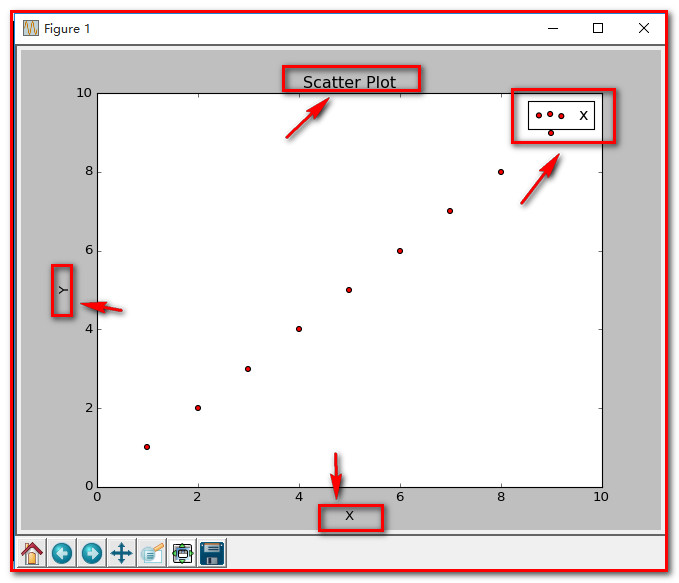5、当scatter后面参数中数组的使用方法，如s，当s是同x大小的数组，表示x中的每个点对应s中一个大小，其他如c，等用法一样，如下：
（1）、不同大小
[python] view plain copy
#导入必要的模块  import numpy as np  import matplotlib.pyplot as plt  #产生测试数据  x = np.arange(1,10)  y = x  fig = plt.figure()  ax1 = fig.add_subplot(111)  #设置标题  ax1.set_title('Scatter Plot')  #设置X轴标签  plt.xlabel('X')  #设置Y轴标签  plt.ylabel('Y')  #画散点图  sValue = x*10  ax1.scatter(x,y,s=sValue,c='r',marker='x')  #设置图标  plt.legend('x1')  #显示所画的图  plt.show()（2）、不同颜色
[python] view plain copy
#导入必要的模块  import numpy as np  import matplotlib.pyplot as plt  #产生测试数据  x = np.arange(1,10)  y = x  fig = plt.figure()  ax1 = fig.add_subplot(111)  #设置标题  ax1.set_title('Scatter Plot')  #设置X轴标签  plt.xlabel('X')  #设置Y轴标签  plt.ylabel('Y')  #画散点图  cValue = ['r','y','g','b','r','y','g','b','r']  ax1.scatter(x,y,c=cValue,marker='s')  #设置图标  plt.legend('x1')  #显示所画的图  plt.show()
结果：（3）、线宽linewidths
[python] view plain copy
#导入必要的模块  import numpy as np  import matplotlib.pyplot as plt  #产生测试数据  x = np.arange(1,10)  y = x  fig = plt.figure()  ax1 = fig.add_subplot(111)  #设置标题  ax1.set_title('Scatter Plot')  #设置X轴标签  plt.xlabel('X')  #设置Y轴标签  plt.ylabel('Y')  #画散点图  lValue = x  ax1.scatter(x,y,c='r',s= 100,linewidths=lValue,marker='o')  #设置图标  plt.legend('x1')  #显示所画的图  plt.show()注：  这就是scatter基本的用法。展开全文python 机器学习 编程语言 散点图
•Python
• ## plt.scatter()

万次阅读 多人点赞 2018-07-31 11:42:23
总是去别人的博客里面找关于scatter散点图相关用法，想想还是自己写一个吧，下次看自己的就行。 函数的原型： matplotlib.pyplot.scatter(x, y, s=None, c=None, marker=None, cmap=None, norm=None, vmin=None, ...
总是去别人的博客里面找关于scatter散点图相关用法，想想还是自己写一个吧，下次看自己的就行。
函数的原型：
matplotlib.pyplot.scatter(x, y, s=None, c=None, marker=None, cmap=None, norm=None, vmin=None, vmax=None, alpha=None, linewidths=None, verts=None, edgecolors=None, *, data=None, **kwargs)
参数的解释：
x，y：表示的是大小为(n,)的数组，也就是我们即将绘制散点图的数据点
s:是一个实数或者是一个数组大小为(n,)，这个是一个可选的参数。
c:表示的是颜色，也是一个可选项。默认是蓝色'b',表示的是标记的颜色，或者可以是一个表示颜色的字符，或者是一个长度为n的表示颜色的序列等等，感觉还没用到过现在不解释了。但是c不可以是一个单独的RGB数字，也不可以是一个RGBA的序列。可以是他们的2维数组（只有一行）。
marker:表示的是标记的样式，默认的是'o'。
cmap:Colormap实体或者是一个colormap的名字，cmap仅仅当c是一个浮点数数组的时候才使用。如果没有申明就是image.cmap
norm:Normalize实体来将数据亮度转化到0-1之间，也是只有c是一个浮点数的数组的时候才使用。如果没有申明，就是默认为colors.Normalize。
vmin,vmax:实数，当norm存在的时候忽略。用来进行亮度数据的归一化。
alpha：实数，0-1之间。
linewidths:也就是标记点的长度。
函数的用法的样例：
1.s和c大小都跟原数组的大小一致。
import numpy as np
import matplotlib.pyplot as plt

np.random.seed(1)
x=np.random.rand(10)
y=np.random.rand(10)

colors=np.random.rand(10)
area=(30*np.random.rand(10))**2

plt.scatter(x,y,s=area,c=colors,alpha=0.5)
plt.show()

结果：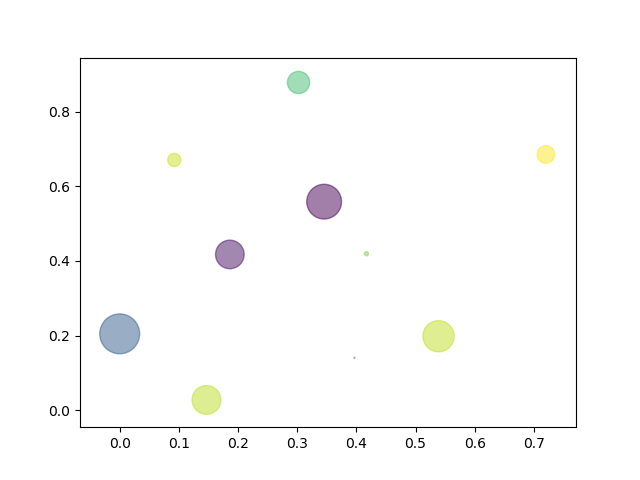2.修改上面的代码的maker，改成x的样本
import numpy as np
import matplotlib.pyplot as plt

np.random.seed(1)
x=np.random.rand(10)
y=np.random.rand(10)

colors=np.random.rand(10)
area=(30*np.random.rand(10))**2

plt.scatter(x,y,s=area,c=colors,alpha=0.5,marker='x')
plt.show()

结果: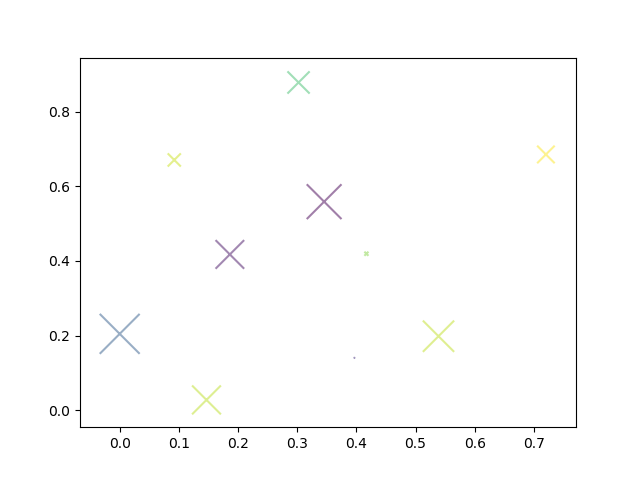3.修改之前的代码的s，表示大小都是一致的。
import numpy as np
import matplotlib.pyplot as plt

np.random.seed(1)
x=np.random.rand(10)
y=np.random.rand(10)

colors=np.random.rand(10)
area=(30*np.random.rand(10))**2
plt.scatter(x,y,s=200,c=colors,alpha=0.5,)
plt.show()

结果：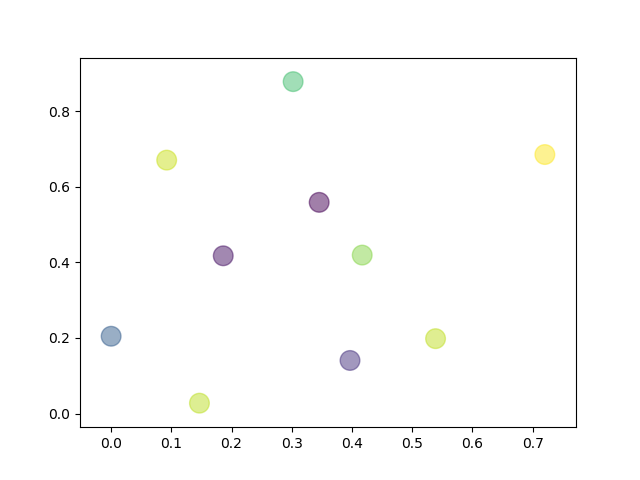4.修改其中的linewidth参数的大小，看到其中的不同了吗？注意只有marker为封闭的图案的时候，这个参数才有效！
import numpy as np
import matplotlib.pyplot as plt

np.random.seed(1)
x=np.random.rand(10)
y=np.random.rand(10)

colors=np.random.rand(10)
area=(30*np.random.rand(10))**2
lines=np.zeros(10)+5
plt.scatter(x,y,s=200,c=
'b',alpha=0.5,linewidths=lines)
plt.show()

结果：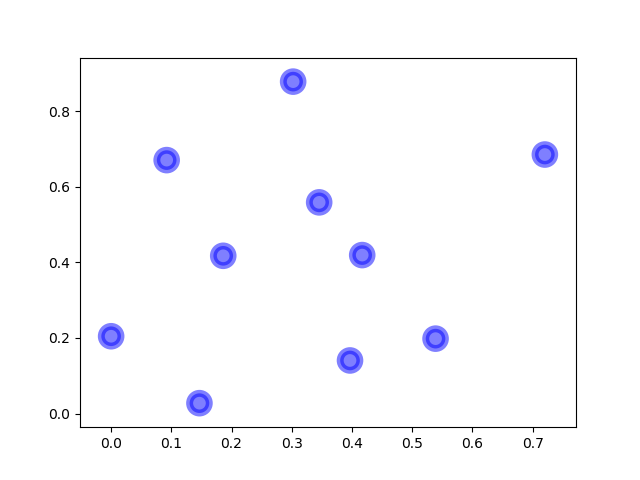展开全文• 转载自https://ww2.mathworks.cn/help/matlab/ref/scatter.html#btrli6p-1scatter散点图语法scatter(x,y)scatter(x,y,sz)scatter(x,y,sz,c)scatter(___,'filled')scatter(___,mkr)scatter(___,Name,Value)scatter(ax...
转载自https://ww2.mathworks.cn/help/matlab/ref/scatter.html#btrli6p-1
scatter

散点图

语法


scatter(x,y)


scatter(x,y,sz)


scatter(x,y,sz,c)


scatter(___,'filled')


scatter(___,mkr)


scatter(___,Name,Value)



scatter(ax,___)



s = scatter(___)


说明


scatter(x,y) 在向量 x 和 y 指定的位置创建一个包含圆形的散点图。该类型的图形也称为气泡图。



scatter(x,y,sz) 指定圆大小。要绘制大小相等的圆圈，请将 sz 指定为标量。要绘制大小不等的圆，请将 sz 指定为长度等于 x 和 y 的长度的向量。



scatter(x,y,sz,c) 指定圆颜色。要以相同的颜色绘制所有圆圈，请将 c 指定为颜色名称或 RGB 三元数。要使用不同的颜色，请将 c 指定为向量或由 RGB 三元数组成的三列矩阵。



scatter(___,'filled') 填充圆形。可以将 'filled' 选项与前面语法中的任何输入参数组合一起使用。



scatter(___,mkr) 指定标记类型。



scatter(___,Name,Value) 使用一个或多个名称-值对组参数修改散点图。例如，'LineWidth',2 将标记轮廓宽度设置为 2 磅。



scatter(ax,___) 将在 ax 指定的坐标区中，而不是在当前坐标区中绘制图形。选项 ax 可以位于前面的语法中的任何输入参数组合之前。



s = scatter(___) 返回 Scatter 对象。在创建散点图后，以后可使用 s 对其进行修改。


输入参数

x - x 值向量

x 值，指定为向量。x 和 y 必须是长度相等的向量。
数据类型： single | double | int8 | int16 | int32 | int64 | uint8 | uint16 | uint32 | uint64 | categorical | datetime | duration

y - y 值向量

y 值，指定为向量。x 和 y 必须是长度相等的向量。
数据类型： single | double | int8 | int16 | int32 | int64 | uint8 | uint16 | uint32 | uint64 | categorical | datetime | duration

sz - 标记面积36 （默认） | 数值标量 | 行或列向量 | []

以平方磅为单位的标记面积，指定为下列形式之一：

数值标量 - 以相同的大小绘制所有标记。行或列向量 - 使每个标记具有不同的大小。x、y 和 sz 中的相应元素确定每个标记的位置和面积。sz 的长度必须等于 x 和 y 的长度。[] - 使用 36 平方磅的默认面积。

标记面积的单位为平方磅。
示例： 50
示例： [36 25 25 17 46]

c - 标记颜色[0 0 1] （默认） | RGB 三元数 | 由 RGB 三元数组成的三列矩阵 | 向量 | 'r' | 'g' | 'b' | ...

标记颜色，指定为下列形式之一：

RGB 三元数或颜色名称 - 使用相同的颜色绘制所有标记。由 RGB 三元数组成的三列矩阵 - 对每个标记使用不同的颜色。矩阵的每行为对应标记指定一种 RGB 三元数颜色。行数必须等于 x 和 y 的长度。向量 - 对每个标记使用不同的颜色，并以线性方式将 c 中的值映射到当前颜色图中的颜色。c 的长度必须等于 x 和 y 的长度。要更改坐标区的颜色图，请使用 colormap 函数。如果散点图中有三个点，并且您希望这些颜色成为颜色图的索引，请以三元素列向量的形式指定 c。

RGB 三元数是包含三个元素的行向量，其元素分别指定颜色中红、绿、蓝分量的强度。强度值必须位于 [0,1] 范围内，例如 [0.4 0.6 0.7]。此外，还可以按名称指定一些常见的颜色。下表列出长和短颜色名称选项以及对应的 RGB 三元数值。

选项说明对应的 RGB 三元数'red' 或 'r'红色[1 0 0]'green' 或 'g'绿色[0 1 0]'blue' 或 'b'蓝色[0 0 1]'yellow' 或 'y'黄色[1 1 0]'magenta' 或 'm'品红色[1 0 1]'cyan' 或 'c'青蓝色[0 1 1]'white' 或 'w'白色[1 1 1]'black' 或 'k'黑色[0 0 0]

示例： 'k'
示例： [1 2 3 4]

mkr - 标记类型'o' （默认） | '+' | '*' | '.' | 'x' | ...

标记类型，指定为下表中列出的值之一。

值说明'o'圆圈'+'加号'*'星号'.'点'x'叉号'square' 或 's'方形'diamond' 或 'd'菱形'^'上三角'v'下三角'>'右三角'<'左三角'pentagram' 或 'p'五角星（五角形）'hexagram' 或 'h'六角星（六角形）'none'无标记

'filled' - 用于填充标记内部的选项'filled'

用于填充标记内部的选项，指定为 'filled'。此选项和具有一个面的标记（例如，'o' 或 'square'）一起使用。没有面而只有边的标记无法填充，如 '+'、'*'、'.' 和 'x'。
'filled' 选项将 Scatter 对象的 MarkerFaceColor 属性设置为 'flat'，并将 MarkerEdgeColor 属性设置为 'none'，这样便可只填充标记的面，而不绘制边。

ax - 目标坐标区Axes 对象 | PolarAxes 对象

目标坐标区，指定为 Axes 对象或 PolarAxes 对象。如果不指定坐标区或当前坐标区是笛卡尔坐标区，scatter 函数将使用当前坐标区。要在极坐标区上绘图，请指定 PolarAxes 对象作为第一个输入参数，或者使用 polarscatter 函数。

名称-值对组参数

示例： 'MarkerFaceColor','red' 将标记面颜色设置为红色。

此处列出的 Scatter 对象属性只是其中的一部分。有关完整列表，请参阅 Scatter 属性。

全部折叠

'MarkerEdgeColor' - 标记轮廓颜色'flat' （默认） | 'none' | RGB 三元数 | 'r' | 'g' | 'b' | ...

标记轮廓颜色，指定为 'flat'、RGB 三元数或表中列出的颜色选项之一。默认值 'flat' 将使用 CData 属性中的颜色。
如果想自定义颜色，请指定一个 RGB 三元数。RGB 三元数是包含三个元素的行向量，其元素分别指定颜色中红、绿、蓝分量的强度。强度值必须位于 [0,1] 范围内，例如 [0.4 0.6 0.7]。此外，还可以按名称指定一些常见的颜色。下表列出长和短颜色名称选项以及对应的 RGB 三元数值。

选项说明对应的 RGB 三元数'red' 或 'r'红色[1 0 0]'green' 或 'g'绿色[0 1 0]'blue' 或 'b'蓝色[0 0 1]'yellow' 或 'y'黄色[1 1 0]'magenta' 或 'm'品红色[1 0 1]'cyan' 或 'c'青蓝色[0 1 1]'white' 或 'w'白色[1 1 1]'black' 或 'k'黑色[0 0 0]'none'无颜色不适用

示例： [0.5 0.5 0.5]
示例： 'blue'

'MarkerFaceColor' - 标记填充颜色'none' （默认） | 'flat' | 'auto' | RGB 三元数 | 'r' | 'g' | 'b' | ...

标记填充颜色，指定为 'flat'、'auto'、RGB 三元数或表中列出的颜色选项之一。

'flat' - 使用 CData 值设置颜色。'auto' - 使用与坐标区的 Color 属性相同的颜色。

如果想自定义颜色，请指定一个 RGB 三元数。RGB 三元数是包含三个元素的行向量，其元素分别指定颜色中红、绿、蓝分量的强度。强度值必须位于 [0,1] 范围内，例如 [0.4 0.6 0.7]。此外，还可以按名称指定一些常见的颜色。下表列出长和短颜色名称选项以及对应的 RGB 三元数值。

选项说明对应的 RGB 三元数'red' 或 'r'红色[1 0 0]'green' 或 'g'绿色[0 1 0]'blue' 或 'b'蓝色[0 0 1]'yellow' 或 'y'黄色[1 1 0]'magenta' 或 'm'品红色[1 0 1]'cyan' 或 'c'青蓝色[0 1 1]'white' 或 'w'白色[1 1 1]'black' 或 'k'黑色[0 0 0]'none'无颜色不适用

示例： [0.3 0.2 0.1]
示例： 'green'

'LineWidth' - 标记边缘的宽度0.5 （默认） | 正值

标记边缘的宽度，指定为一个正值（以磅为单位）。
示例： 0.75

输出参数

全部折叠

s - Scatter 对象Scatter 对象

Scatter 对象。在创建散点图后，可使用 s 访问和修改散点图的属性。

扩展功能

tall 数组对行数太多而无法放入内存的数组进行计算。

示例

创建散点图

创建 x 为 0 和之间的 200 个等间距值。创建 y 为带随机干扰的余弦值。然后，创建一个散点图。


x = linspace(0,3*pi,200);
y = cos(x) + rand(1,200);
scatter(x,y)改变圆圈大小

使用大小不同的圆圈创建一个散点图。以平方磅为单位指定大小


x = linspace(0,3*pi,200);
y = cos(x) + rand(1,200);
sz = linspace(1,100,200);
scatter(x,y,sz)x、y 和 sz 中的相应元素确定每个圆圈的位置和大小。要按照相同的面积绘制所有圆圈，请将 sz 指定为数值标量。

改变圆圈颜色

创建一个散点图并改变圆圈的颜色。


x = linspace(0,3*pi,200);
y = cos(x) + rand(1,200);
c = linspace(1,10,length(x));
scatter(x,y,[],c)x、y 和 c 中的相应元素确定每个圆形的位置和颜色。scatter 函数将 c 中的元素映射到当前颜色图中的元素。

填充标记

创建一个散点图并填充标记。scatter 使用标记边缘的颜色填充每个标记。


x = linspace(0,3*pi,200);
y = cos(x) + rand(1,200);
sz = 25;
c = linspace(1,10,length(x));
scatter(x,y,sz,c,'filled')指定标记符号

创建向量 x 和 y，作为带随机干扰的正弦和余弦值。然后，创建一个散点图，并使用面积为 140 平方磅的菱形标记。


theta = linspace(0,2*pi,150);
x = sin(theta) + 0.75*rand(1,150);
y = cos(theta) + 0.75*rand(1,150);
sz = 140;
scatter(x,y,sz,'d')更改标记颜色和线条宽度

创建向量 x 和 y，作为带随机干扰的正弦和余弦值。创建一个散点图并设置标记边缘颜色、标记面颜色和线条宽度。


theta = linspace(0,2*pi,300);
x = sin(theta) + 0.75*rand(1,300);
y = cos(theta) + 0.75*rand(1,300);
sz = 40;
scatter(x,y,sz,'MarkerEdgeColor',[0 .5 .5],...
'MarkerFaceColor',[0 .7 .7],...
'LineWidth',1.5)指定散点图的子图

创建一个包含两个子图的图窗，并向每个子图添加一个散点图。对下部子图中的散点图使用填充的菱形标记。


x = linspace(0,3*pi,200);
y = cos(x) + rand(1,200);
ax1 = subplot(2,1,1);
scatter(ax1,x,y)

ax2 = subplot(2,1,2);
scatter(ax2,x,y,'filled','d')创建并修改散点序列

创建一个散点图并返回散点序列对象 s。


theta = linspace(0,1,500);
x = exp(theta).*sin(100*theta);
y = exp(theta).*cos(100*theta);
s = scatter(x,y);在创建散点序列后，使用 s 查询并设置其属性。将线宽设置为 0.6 磅。将标记边缘颜色设置为蓝色。使用 RGB 三元数颜色设置标记面。
注意：从 R2014b 开始，您可以使用圆点表示法设置属性。如果您正在使用早期版本，请改用 set 函数，例如 set(s,'LineWidth',0.6)。


s.LineWidth = 0.6;
s.MarkerEdgeColor = 'b';
s.MarkerFaceColor = [0 0.5 0.5];展开全文matlab
• 分散走 用于Scatter的Golang驱动程序遵循scatter-js的方式开发Go
• scatter方法通过src和index两个张量来获得一个新的张量。 torch_scatter.scatter(src: torch.Tensor, index: torch.Tensor, dim: int = - 1, out: Optional[torch.Tensor] = None, dim_size: Optional[int] = None, ...python
• 函数原型:matplotlib.pyplot.scatter(x, y, s=None, c=None, marker=None, cmap=None, norm=None,vmin=None, vmax=None, alpha=None, linewidths=None,verts=None, edgecolors=None, hold=None, data=None,**kwargs)...
• In addition, we provide the following composite functions which make use of scatter_* operations under the hood: scatter_std, scatter_logsumexp, scatter_softmax and scatter_log_softmax. All included ...
• 详细 分析了scatter 文件的各种语法使用格式和方法功能。
• 进入python3，遇到这个错误 python import torch_scatter Traceback (most recent call last): File "", line 1, in File "/home/dell/.local/lib/python3.6/site-packages/torch_scatter/__init__.py", line 3, ...pytorch
• torch_scatter.scatter_add 官方文档：torch_scatter.scatter_add(src,index,dim=-1,out=None,dim_size=None,fill_value=0) Sums all values from thesrctensor intooutat the indices specified in theinde...pytorch
• ## pytorch中scatter()、scatter_()详解

千次阅读 多人点赞 2020-01-07 11:41:27
scatter()、scatter_() scatter() 和 scatter_() 的作用一样。 不同之处在于 scatter() 不会直接修改原来的 Tensor，而 scatter_() 会在原来的基础上对Tensor进行修改。 torch.scatter()官方文档 scatter(dim, index...pytorch
• 1、scatter函数原型 2、其中散点的形状参数marker如下： 3、其中颜色参数c如下:
• from torch_scatter import scatter_max x, _ = scatter_max(x, data.batch, dim=0) 例子 from torch_scatter import scatter_max import torch if __name__ == "__main__": t1 = torch.tensor([[0, 2], [2, 2...
• scatter()、scatter_() scatter() 和 scatter_() 的作用一样。 不同之处在于 scatter() 不会直接修改原来的 Tensor，而 scatter_() 会在原来的基础上对Tensor进行修改。 torch.scatter()官方文档 scatter(dim, index...
• 根据映像文件中地址映射的复杂程度，有两种方法来告诉ARM链接器这些相关的信息。对于映像文件中地址映射关系比较简单的情况，可以使用命令行选项；...Scatter文件又称为分散加载文件，将重点讲解如何编写scatter文件。
• 300) colors1 = '#00CED1' #点的颜色 colors2 = '#DC143C' area = np.pi * 4**2 # 点面积 # 画散点图 plt.scatter(x1, y1, s=area, c=colors1, alpha=0.4, label='类别A') plt.scatter(x2, y2, s=area, c=colors2, ...
• scatter() 和 scatter_() 的作用是一样的，只不过 scatter() 不会直接修改原来的 Tensor，而 scatter_() 会PyTorch 中，一般函数加下划线代表直接在原来的 Tensor 上修改scatter(dim, index, src) 的参数有 3 个dim...
•   最近开始学习Python编程，遇到scatter函数，感觉里面的参数不知道什么意思于是查资料,最后总结如下...
• eosforce 使用 scatter API 1. 安装 git clone https://github.com/eosforce/scatter_demo.git cd scatter_demo npm install 2. 运行 npm run dev 配置eosforce 到sccater 进入scater设置>网络，然后选择新建，选择...JavaScript
• 列出scatter常用的一些参数： plt.scatter(x,y,c= '颜色可选',marker= '点的样式', cmap= '颜色变化',alpha=“透明度”, linewidths=“线宽”,s= '点的大小')其中 颜色可选：b--blue c--cyan g--green k--blackm--...
• scatter() 和 scatter_() 的作用是一样的，只不过 scatter() 不会直接修改原来的 Tensor，而 scatter_() 会 PyTorch 中，一般函数加下划线代表直接在原来的 Tensor 上修改 scatter(dim, index, src) 的参数有 3 个 ...
• 文章目录scatter` scatter 用一张官网的图 import torch from torch_scatter import scatter index = torch.tensor([0,0,1,0,2]) input = torch.tensor([[1,1],[1,1],[2,2],[1,1],[1,1]]) result = scatter(input......